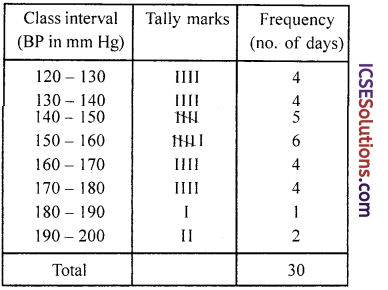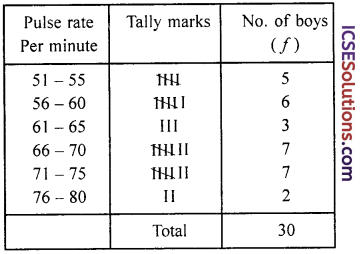Well-structured S Chand Class 9 Maths Solutions ICSE Chapter 14 Statistics, Introduction, Data and Frequency Distribution Ex 14 facilitate a deeper understanding of mathematical principles.

## S Chand Class 9 ICSE Maths Solutions Chapter 14 Statistics, Introduction, Data and Frequency Distribution Ex 14

Question 1.
Fill in the blanks :
(i) The difference between the maximum and the minimum observations in a set of data is ………….. called the of the data.
(ii) The number of observations in a particular class interval is called the ………….. of the class interval.
Solution:
(i) The difference between the maximum and the minimum observations in a set of data is called the range of the data.
(ii) The number of observations in a particular class interval is called the frequency of the class interval.

Question 2.
Fill in the blanks :
(i) Lower limit of the class interval 26 – 33 is …………..
(ii) Upper limit of the class interval 21 – 25 is …………..
(iii) The class mark of the class interval 20 – 29 is …………..
(iv) The class mark of the class interval 9.5 – 19.5 is …………..
(v) If the class-marks of a distribution are 32, 39, 46, 53; then the width of each class interval is …………..
(vi) The mid-point of a class interval is called its …………..
(vii) If the class marks of a distribution are 28, 34, 40, 46, 52; then the class intervals are of length …………..
(viii)If the class marks in a frequency distribution are 19.5, 26.5, 33.5, 40.5 47.5, 54.5, 61.5, then the class size of the distribution is ………….
Solution:
(i) Lower limit of the class interval 26 – 33 is 26.
(ii) Upper limit of the class interval 21 – 25 is 25.
(iii) The class mark of the class interval 20 – 29 is $$\frac{20+29}{2}=\frac{49}{2}$$ = 24.5.
(iv) The class mark of the class interval 9.5 – 19.5 is $$\frac{9.5+19.5}{2}=\frac{29}{2}$$ = 14.5.
(v) If the class-marks of a distribution are 32, 39, 46, 53; then the width of each class interval is C.I. = 39 – 32 = 7.
(vi) The mid-point of a class interval is called its class mark.
(vii) If the class marks of a distribution are 28, 34, 40, 46, 52; then the class intervals are of length C.I. = 34 – 28 = 6.
(viii) If the class marks in a frequency distribution are 19.5, 26.5, 33.5, 40.5 47.5, 54.5, 61.5, then the class size of the distribution is class size = 26.5 – 19.5 = 7.Question 3.
Fill in the blanks :
(i) The range of the set of data 5, 8, 15, 21, 7, 10 is …………..
(ii) The range of the set of data 15, 13, 14, 17, 19, 16, 14, 15 is …………..
(iii) The class mark of the class interval 10 – 19 is …………..
(iv) The class mark of the class interval 9.5 – 16.5 is …………..
Solution:
(i) The range of the set of data 5, 8, 15, 21,7, 10 is 10 – 5 = 5.
(ii) The range of the set of data 15, 13, 14, 17, 19, 16, 14, 15 is 19 – 14 = 5.
(iii) The class mark of the class interval 10 – 19 is c.m, of 10 – 19 = $$\frac{19+10}{2}=\frac{29}{2}$$ = 14.5.
(iv) The class mark of the class interval 9.5 – 16.5 is c.m. of 9.5 – 16.5 = $$\frac{9.5+16.5}{2}$$ = $$\frac { 26 }{ 2 }$$ = 13.

Question 4.
Which of the following variables are continuous and which are discrete :
(a) size of shoes,
(b) number of pages in a book,
(c) distance travelled by a train,
(d) time,
(e) daily temperature,
(f) intelligence quotient,
(g) number of goals scored in a hockey-match,
(h) height,
(i) weight,
(j) number of rooms in apartments,
(k) number of wage earners in a factory,
(l) number of car accidents.
Solution:
We know that a variable which can theoritically assume any value between two given values is continuous otherwise it is discrete. Therefore
(a) size of shoes – discrete
(b) number of pages in a book – continuous
(c) distance travelled by a train – continuous
(d) time – continuous
(e) daily temperature – continuous
(f) intelligence quotient – continuous
(g) number of goals scored in a hockey-match – discrete
(h) height – continuous
(i) weight – continuous
(j) number of rooms in appartments – discrete
(k) number of wage earners in a factory – discrete
(l) number of car accidents – discreteQuestion 5.
The class marks of a distribution are 2.03, 2.23, 2.43, 2.63, 2.83, 3.03 and 3.23 Determine the class size and the class boundaries.
Solution:
The class marks are 2.03, 2.23, 2.43, 2.63, 2.83, 3.03 and 3.23
Then class size = difference of two consecutive class marks
= 2.23 – 2.03 = 0.20
∴ Class boundries will be
2.03 – $$\frac { 0.20 }{ 2 }$$ = 2.03 – 0.10 = 1.93
and 2.03 + $$\frac { 0.20 }{ 2 }$$ = 2.03 + 0.10 = 2.13
∴ 1.93 – 2.13
Similarly other boundries will be
2.13 – 2.33, 2.33 – 2.53, 2.53 – 2.73,
2.73 – 2.93, 2.93 – 3.13 and 2.13 – 2.33

Question 6.
The following are the monthly rents (in rupees) of 30 shops :
42, 49, 37, 82, 37, 75, 62, 54, 79, 84, 75, 63, 44, 74, 44, 36, 69, 54, 48, 74, 39, 48, 45,61,71,47,38, 80,51,31.
Using the class intervals of equal width in which one class interval being 40-50 (excluding 50). construct a frequency table for the above data.
Solution:
Highest rent = 84
Lowest rent = 31
∴ Range = 84 – 31 = 53
Now taking class intervals 40 – 50 (excluding 50)Question 7.
Construct a frequency table for the following set of data of weights (in gms) of 30 oranges using equal class intervals one of them being 50-60 (60 not included).
45, 55, 30, 85, 75, 85, 40, 60, 65, 40, 60, 75, 70, 60, 70, 85, 85, 80, 35, 45, 40, 50, 60, 65, 55, 45, 30, 80, 85, 75.
Solution:
Greatest weight = 85
Lowest weight = 30
Range = 85 – 30 = 55
Taking class intervals such as 50 – 60, (60 not included)Question 8.
Given below are the Systolic Blood Pressure readings (mm Hg.) made on a person for 30 days. Construct a frequency distribution for the data using class intervals of equal size, one of them being 150-160 (160 not included).
165, 159, 190, 150, 171, 182, 165, 141, 135, 155,192,152,155,145, 145,160, 175, 179, 178, 120,125, 128, 152, 135, 142, 128, 140, 130, 130, 160.
Solution:
Highest BP = 192
Lowest BP = 120
Range = 192 – 120 = 72
Taking class intervals as 150-160 (160 not included)Question 9.
Present the following data of the %marks of 60 students in the form of a frequency table with 10 classes of equal width, one class being 40-49.

 41 17 83 63 54 92 60 58 70 06 67 82 30 44 57 49 34 73 54 63 36 52 32 75 60 38 09 79 28 30 42 93 43 80 03 32 57 67 24 64 63 11 35 82 10 38 00 41 60 32 72 53 92 88 62 55 60 33 40 57

Solution:
Highest percentage of marks = 93
Lowest percentage = 03
Range = 93 – 03 = 90
Taking class intervals as 40 – 49Question 10.
Thirty 16 year old boys were tested to find their pulse rate. This following figures were obtained for the number of beats per minute. Using the class intervals 51-55, 56-60, etc., of equal width, prepare a frequency table.
55, 72, 70, 66, 74, 70, 74, 53, 57, 62, 71, 58, 68, 75, 79, 68, 63, 59, 54, 51, 61, 66, 78, 73, 59, 52, 66, 60, 72, 56.
Solution:
Highest pulse rate = 79
Lowest pulse rate = 51
Range = 79 – 51 = 28
Using the class intervals like 51 – 55, 56 – 60 etc.